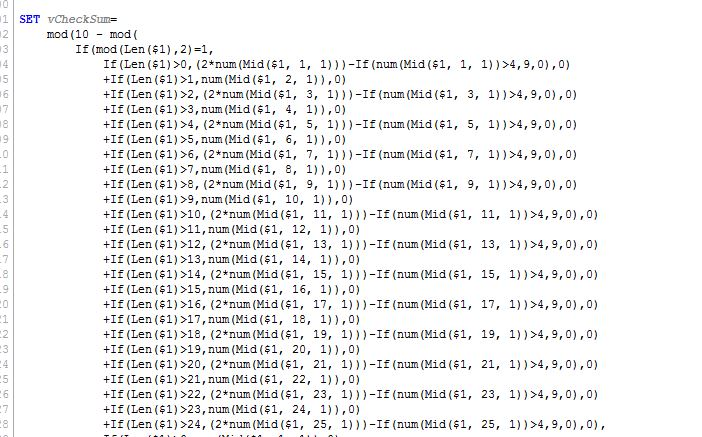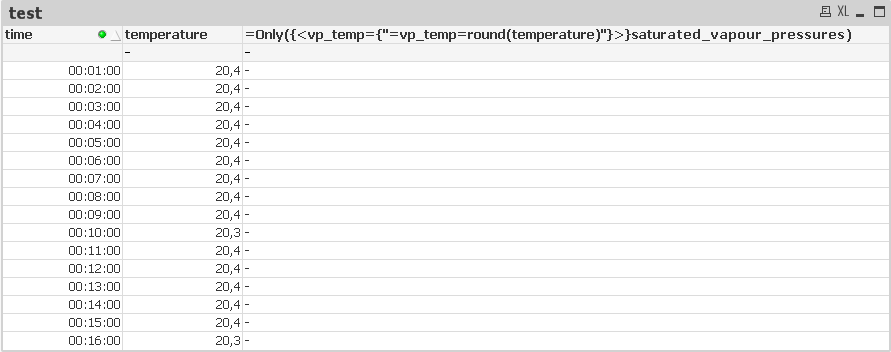# New to QlikView

Discussion board where members can get started with QlikView.

Announcements
Leverage your QlikView investment to modernize BI – see how! Join Group
cancel
Showing results for
Did you mean:
HighlightedNot applicable

## user defined function to select values from a table for subsequent calculation

Hey,

I use this user defined function to calculate the water content of air. :

0.622 * (rel. hum * saturated vapour pressure) / (air pressure - rel. hum * saturated vapour pressure)

-->

SET udf_water_content = Num(0.622*(\$1*\$2)/((\$3-\$1*\$2)));

But now I would like to get the saturated vapour pressure of the measured temperature instead of a fix value.

water_content = \$(udf_water_content(humidity,=\$(VapPress(=\$(round(temperature))),101300));

I have a table with temperatures from -20 to 99 °C with the associated saturated vapour pressures. So how can I define the VapPress function to select the vapour pressure of the associated temperature? Attached you can see an extract of the table with the preassures. The meassured temperatures are floats, so I would need to round them as well.

temp
saturated_vapour_pressures
171936,2
182062
192196
202337
212485
222642

Thanks for feedback.

BR

Henrik

Tags (6)
14 Replies
HighlightedSpecialist

## Re: user defined function to select values from a table for subsequent calculation

You can embed If statements (and I guess other functions) in user defined functions. That said it did take me a while to get the brackets right on this one;HighlightedMVP & Luminary

## Re: user defined function to select values from a table for subsequent calculation

The expression to select pressure for a specific temp would be:

Only({<temp={18}>}saturated_vapour_pressures)

and for a rounded value

Only({<temp={\$(=round(18.2))}>}saturated_vapour_pressures)

So the function version would be:

Only({<temp={\$(=round(\$1))}>}saturated_vapour_pressures)

The \$() is going to give you some grief if you try to define as is with a SET statement. One workaround is to escape the \$ using replace like this:

LET VapPress3 = replace(

'Only({<temp={@(=round(\$1))}>}saturated_vapour_pressures)'

,'@','\$');

Another alternative to use another expression that doesn't use \$().

SET VapPress = Only({<temp={"=temp=round(\$1)"}>}saturated_vapour_pressures);

-Rob

http://robwunderlich.com

HighlightedNot applicable

## Re: user defined function to select values from a table for subsequent calculation

Hey Rob,

first of all I would like to thank you for your detailed reply! But I still got one issue. When I use your function to select the associated saturated vapour pressure it works with a manual entered temperature. It works as well when I insert the function in my first function.

Functions

SET udf_wc = Num(0.622*((\$1*\$2)/((\$3-\$1)*\$2)));

SET udf_svp = Only({<vp_temp={"=vp_temp=round(\$1)"}>}saturated_vapour_pressures);

Correct result with following functions

=\$(udf_svp(18.2))

=\$(udf_wc(50,\$(udf_svp(18.2)),101300))

But now it comes to diagramms. I use a table with measured data containing the columns "time", "temperature" and, "humidity".

If I choose "time" as the dimension, I can use the function "=\$(udf_wc(humidity,2337,101300))" correctly. It also works, when I do this: "=\$(udf_wc(humidity,\$(udf_svp(20)),101300))". But if I try this "=\$(udf_wc(humidity,\$(udf_svp(temperature)),101300))" it doesn't work. But I absolutely have no clue why.

Thanks for any help!

BR

Henrik

HighlightedMVP & Luminary

## Re: user defined function to select values from a table for subsequent calculation

There has to be a single temperature value for the row. If not, some type of aggregation function needs to be used like:

=\$(udf_wc(humidity,\$(udf_svp(max(temperature))),101300))

Could that be the issue?

-Rob

HighlightedNot applicable

## Re: user defined function to select values from a table for subsequent calculation

hey rob,

there exists a single temperature value for every row. Same as for humidity. So I don't get why it isn't workingIf I use the max() function, all of the calculation works with one temperature value (in my case 21°C). So the diagramm result shows the same curve as the rel. humidity curve and that is not correct.

br

henrik

HighlightedMVP & Luminary

## Re: user defined function to select values from a table for subsequent calculation

It sounds like you have more than one temp per time, or that time snd temp are not properly associated. Can you post a sample qvw?

Rob

HighlightedNot applicable

## Re: user defined function to select values from a table for subsequent calculation

Hey rob,

I am 100% sure I have a temp per time. For example if I do following it shows a curve as well:

=\$(udf_wc(temperature,\$(udf_svp(20,1)),101300))

Only in combination of both with time associated values it doesn't work ...

=\$(udf_wc(humidity,\$(udf_svp(temperature)),101300))

Ah and I just recently figgured out that it also don't work if I do following:

=\$(udf_wc(20,\$(udf_svp(humidity)),101300))

So the bug must be somewhere in the function "udf_svp" ... just for the records, I used this one (my columns names are "vp_temp" and "saturated_vapour_pressures":

SET udf_svp = Only({<vp_temp={"=vp_temp=round(\$1)"}>}saturated_vapour_pressures);

BR

Henrik

HighlightedMVP & Luminary

## Re: user defined function to select values from a table for subsequent calculation

Change your chart type to a Straight Table and use an empty label. You should be able to see the substituted formulas in the headings. You can try it piece by piece.

-Rob

HighlightedNot applicable

## Re: user defined function to select values from a table for subsequent calculation

I tried ... this is the result ... can't figure out why it isn't working: